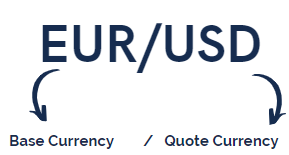# How can I calculate 1 pip of profit or loss?

Please use the calculation formula below to calculate the pip value.

Calculation Formula:

Amount of Base Currency * Pips= Value in Quote CurrencyExamples:
Value of 1 pip in EUR/USD= 1 Lot (100 000 €)*0.0001= 10 USD
Value of 1 pip in USD/CHF= 1 Lot (100 000 \$)*0.0001=10 CHF
Value of 1 pip in EUR/JPY=1 Lot (100 000 €)*0.01= 1000 JPY